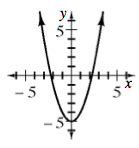Home > INT3 > Chapter 1 > Lesson 1.2.2 > Problem1-89

1-89.

If $g(x) = x^2 - 5$, where does the graph of $g(x)$ cross the $x$-axis? Make a sketch of the graph. Homework Help ✎

Remember problem 1-71.

To find the x-intercepts, or roots, set the equation equal to 0.

$(0) = x^2 - 5$

Solve for $x$.

$5=x^2 \rightarrow \pm \sqrt{5}=x$

$\left(\pm \sqrt{5},0\right)$